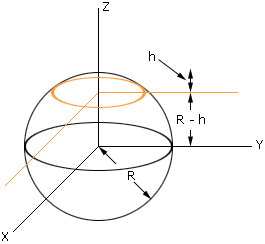Subject: Surface Area Name: Evie Who is asking: Other Level: All Question: I would like to know how to calculate the surface area of a contact lens with a radius of 7mm? I know that the area of a circle is pi R squared, but a contact lens has a dome. Hi Evie, To find the surface area you would have to know the exact shape of the lens. If you knew the height of the dome you might make an approximation by assuming that the lens is a piece of a sphere, but I have no idea how reasonable such an approximation would be. Harley Thanks for your response. So what you are saying is we cannot calculate the surface area without knowing the height of the lens dome? If we do approximate its height, what formula would we use for our calculation of surface area? Evie Suppose that you have a sphere of radius R with center at the origin in 3-space, as in the diagram. Imagine that you slice through the sphere with a plane, parallel to the XY-plane, to cut off a cap with a circular base (orange in the diagram). This cap is the lens.The equation of the sphere is x2 + y2 + z2 = R2 where R is the radius. If the height of the cap is h mm then the circular base has equation x2 + y2 + (R - h)2 = R2 or x2 + y2 = 2Rh - h2 Thus the radius of the circular base of the cap is the square root of 2Rh - h2. In your example this radius is 7mm so 2Rh - h2 = 72, and hence if you know h you can find R. Using calculus you can show that the surface area of the cap is 2*pi*R*h mm2. (* is multiplication.) The problem with this method is that the lens may not be a piece of a sphere. Cheers, Harley Go to Math Central